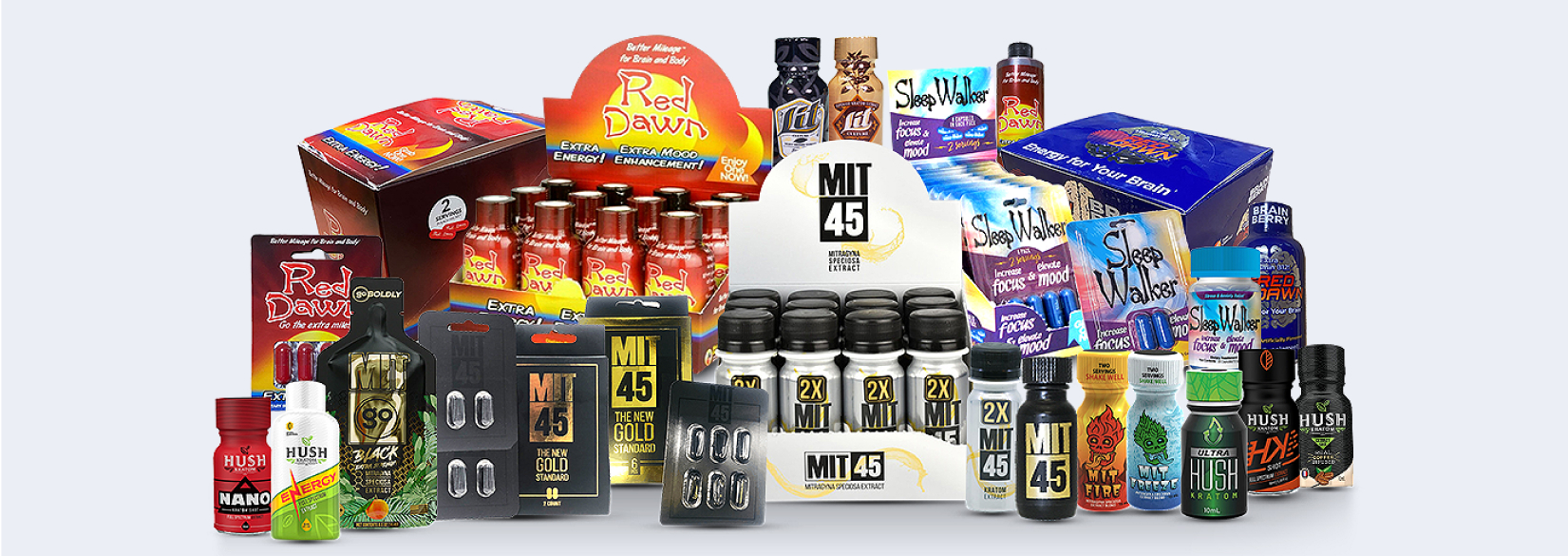# Case Study

## Xtreme Supplements LLCAt Xtreme Supplements, our mission is to provide the best supplements and formulas at unbeatable prices, delivered fast.## Problem

Here is where you add the problems. Here is where you add the problems. Here is where you add the problems. Here is where you add the problems. Here is where you add the problems. Here is where you add the problems. Here is where you add the problems.## Solution

Here is where you add the problems. Here is where you add the problems. Here is where you add the problems. Here is where you add the problems. Here is where you add the problems. Here is where you add the problems. Here is where you add the problems.## Outcome

Here is where you add the problems. Here is where you add the problems. Here is where you add the problems. Here is where you add the problems. Here is where you add the problems. Here is where you add the problems. Here is where you add the problems.## Website Design and Development

You can add the contents for this project here.

### Logo

You can add the contents for this project here. You can add the contents for this project here. You can add the contents for this project here. You can add the contents for this project here.

You can add the contents for this project here. You can add the contents for this project here. You can add the contents for this project here. You can add the contents for this project here.## Social Media Posting

You can add the contents for this project here. You can add the contents for this project here: